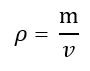# Density Calculator

Easily calculate the density of a substance given its mass and volume. Input supports metric and imperial measurement units. This density calculator can also be used to solve for mass or volume.

Share calculator:

Embed this tool:
get code

## Using the density calculator

The density calculator calculates the volumetric mass density of a substance: the mass concentrations of a pure substance. It should be differentiated with other kinds of density e.g. number density: the particles per unit volume. Mass density is defined as the ratio of the substance's volume to its mass.

The calculator can also be used to solve for any of the other values: mass and volume. When solving for mass you need to know volume and density while when solving for volume you need to know the substance's mass and density.

The output if calculating density is in grams per cm3 or kg per m3, as well as in oz/cu in, lb/cu ft and lb per cubic yard. Grams or kilograms per litre are also available. When the output is mass or volume it is in both standard metric units and imperial ones, with the units automatically being adjusted depending on how big or small the resulting value is.

## Density Formula

The formula for density (ρ - Greek lower-case letter rho) expressed in terms of the body's volume (v) and its mass (m) is the equation:Solving for mass or volume requires straightforward transformations which we used in constructing the density calculator above. The formula is valid for homogenous materials where the density of all points in the object equals its total mass divided by its total volume. Determining the density of a gas or liquid may require the use of specialized equipment like a hydrometer, a dasymeter or a Coriolis flow meter. If the object is of heterogeneous density a piece-wise approach may be preferred.

One should further take into account that this formula is not applicable if the measured volume contains voids (air, vacuum, liquid, gas or solid other than the substance of interest) the calculation would instead result in "bulk density" and not volumetric mass density. Empirical or geometrical estimation of the void fraction of the volume can be performed in order to arrive at the volume of the substance itself. These are obviously not supported by our tool.

## How to find density? Calculation examples

Example 1: If 10 m3 of water at 0 degrees Celsius and at 1 atm pressure weights 9998.395 kg, what is the density of water under these conditions? Using the density equation above we replace the values for m and v and get Density = 9988.395 kg / (10 m3) = 999.8395 kg/m3.

Example 2: If the density of concrete is 2,400 kg/m3 and we have 50 cubic meters of concrete, how much should it weigh, approximately? Since ρ = m / v then m = ρ · v so the mass of the concrete will be 2400 · 50 = 120,000 kg or 120 tonnes. Such calculations are necessary in construction projects when one asks questions such as "how much concrete do I need?", "how much sand do I need?", "how much gravel do I need?" and so on.

Our calculator is especially useful if the input units for the calculation are different than the desired output units, in which case it will perform these unit conversions for you. For example, you would not need a separate volume converter.

## Density of water and other gases & liquids

The density of a material or substance varies with its temperature and pressure, which is typically negligible for solids and liquids but is much greater for gases. Increasing the pressure on a substance decreases its volume and increases its density. Increasing the temperature generally decreases the material density and increases its volume (increasing denominator in the formula). A substance with uneven temperature will also have varying pressure and density. In this sense the density calculation in our tool is an average density.

As an example of how water density changes with temperature, at 1 atm pressure water has an estimated density of 983.854 kg/m3 at -30 °C, 999.9720 kg/m3 at 4 °C and only 958.4 kg/m3 at 100 °C (boiling temperature at sea-level). The density of air at sea level is approximately 1.293 kg/m3 at 0°C, but 1.423 kg/m3 at -25 °C and 1.146 kg/m3 at 35 °C. This shows the need for average density calculations at varying temperatures.

Some of the least dense substances (at 0.987 atm and 0.00 °C) are Hydrogen (0.0898 kg/m3) and Helium (0.179 kg/m3). Water is relatively dense at ~1,000 kg/m3 while metals generally have much higher densities e.g. Iron at 7,870 kg/m3, Gold at 19,320 kg/m3 and Osmium at 22,570 kg/m3.